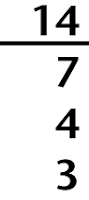## Wednesday, November 25, 2009

I'm not talking about A D D, Attention Deficit Disorder, but the verb add, as in to combine numbers.

I hope not. I think not. How about a quiz today, while we all wait for Thanksgiving to arrive!

First, a bit of review:

Word/Concept Meaning
Count; Counting Repeatedly adding one to a set of numbers; ascending
Plus Added to; this symbol "+"; slightly above normal (B+)
Sum; Summation Calculating the total of two or more numbers
Subtraction; Subtract The inverse of addition; taking one number away from another number

Math words in English are derived from Latin roots.

When adding, the number you start with was first known as the augend. (We use this same root word when we talk about somethings being augmented...)

The word augend means "thing to be increased"
The word add means "give to"The word sum means "the highest" which leads to an interesting fact:

Did you know?

People used to add columns of numbers upwards? Like this:

Did you know addition can be very tricky?

Measurements in different units are tough, as in (3 feet + 4 yards) = 180 inches

Did you know there are things you cannot add?

Different kinds of objects (3 squares plus 4 rectangles) ≠ 7 octagons

Did you know you can be asked to consider two opposite operations at once?

The speed of the car is controlled at 45 mph ±5 mph.

Did you know the secrets of adding Roman numerals?

First, change negatives to positives ( IX means -1 and 10, so you make it V I I I I instead )
Rearrange all the letters in order of highest value to lowest, then simplify the total.
(XIII + III + L + VII  ) = L + X + V + I I I I I I I I = LXXIIIDid you know you can "show" addition on a number line?

Here's an example.

For more on number lines, read our May 2 bog post, "Using Numer Tines to Add and Subtract" and download a free math worksheet.

Did you know about infix notation?

We indicate addition with a plus sign between two numbers (2+2). This is called infix notation.

Did you know addition can be performed in various ways on a calculator or in programs?

Infix notation or direct algebraic notation is when you press 2 + 2 + 2 = and you see 6
Prefix or Polish notation is when you press +  then 2 ↵ 2 ↵ 2 ↵ and the display shows 6
Postfix or Reverse Polish notation is when you press 2 ↵ 2 ↵2  + and the display shows  6

Did you know about operands and operators?

Addition is an operation (something that is performed). The two (or more) addends are also known as  operands (the things upon which a math operation is performed).

Did you know the four special properties of addition?
• commutative - the order of the addends doesn't make any difference in the sum. 2+3=3+2
• associative - when three or more numbers are added, grouping them in different ways doesn't make any difference in the sum (2+3)+4 = 2+(3+4)
• additive identity - the sum of zero and a number is that number. 5+0=5
• distributive property - the sum of two numbers when multiplied by a third is the same as each of the two numbers multiplied independently and then added (3+4)*5=35 and (3*5)+(4*5)=35